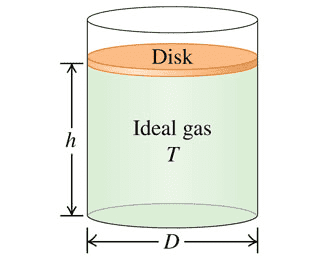# Isothermal Compression of a Ideal Gas and Distance

• elements
Again, please do this algebraically. What do you get for the inside gas pressures...and the final distance the piston will rest?f

## Homework Statement

The figure at the bottom shows a cylindrical tank of diameter D with a moveable 3.00-kg circular disk sitting on top. The disk seals in the gas inside but is able to move without friction. The gas inside is at temperature T. The height of the disk is initially at h = 4.00 m above the bottom of the tank. When a lead brick of mass 9.00 kg is gently placed on the disk, it moves downward, compressing the gas and decreasing the volume. Assuming the temperature of the gas is held constant, at what distance from the bottom of the will the disk come to rest?mass_{disk}=3.00 kg
mass_{Pb}=9.00 kg
m_{T}=12.00kg
h_i=4.00m
Isothermal \therefore T_i=T_f
V_i > V_f ; P_i<P_f

## Homework Equations

\P = \rho*\g*\h
P_1*V_1*T_2=P_2*V_2*T_1
W=nRT*ln(\frac{V_f}{V_i})=m*g*d

## The Attempt at a Solution

\[/B]delta P = -\rho*g*\int_{y}^{4} = -0.5*\rho*g*(y^2-16)
F_{disk+Pb}=(m_{d}+m{Pb})/g=127.53N
P_1*V_1=P_2*V_2
P_1*A_1*H_1=P_2*A_2*H_2 (A_1=A_2)
H_2=4*\frac{P_1}{P_2}

I'm not really sure where to proceed here since the initial pressure nor the final pressure nor any of the volumes are given so I'm generally extremely confused on how to proceed to find the final distance the piston would rest at. I know since that by Boyles law as Volume decreases, Pressure increases at constant Temperature. I'm just not sure how we factor any of that in without being given volume or area.

#### Attachments

I haven't spent the time to work out the solution but I note that Pressure = Force/Area so my guess would be that the area cancels at some point.

Algebraically, in terms of mdisk and g, what is the force of the gas on the disk initially? Algebraically, in terms of mdisk, g, and A (where A is the area of the disk), what is the pressure of the gas P1 on the disk initially?

Algebraically, in terms of mdisk, mPb, and g, what is the force of the gas on the disk finally? Algebraically, in terms of mdisk, mPb, g, and A (where A is the area of the disk), what is the pressure of the gas P2 on the disk finally?

Algebraically, what is the ratio of P1 to P2?

•CWatters
I've got this but I'm not sure how to proceed from here without being given any of the pressures.

$$\delta P = -\rho*g*\int_{y}^{4} = -0.5*\rho*g*(y^2-16)$$
$$F_{disk+Pb}=(m_{d}+m{Pb})/g=127.53N$$
$$P_1*V_1=P_2*V_2$$
$$P_1*A_1*H_1=P_2*A_2*H_2 (A_1=A_2)$$
$$H_2=4*\frac{P_1}{P_2}$$

I've got this but I'm not sure how to proceed from here without being given any of the pressures.

$$\delta P = -\rho*g*\int_{y}^{4} = -0.5*\rho*g*(y^2-16)$$
$$F_{disk+Pb}=(m_{d}+m{Pb})/g=127.53N$$
$$P_1*V_1=P_2*V_2$$
$$P_1*A_1*H_1=P_2*A_2*H_2 (A_1=A_2)$$
$$H_2=4*\frac{P_1}{P_2}$$

Algebraically, in terms of mdisk and g, what is the force of the gas on the disk initially? Algebraically, in terms of mdisk, g, and A (where A is the area of the disk), what is the pressure of the gas P1 on the disk initially?

Algebraically, in terms of mdisk, mPb, and g, what is the force of the gas on the disk finally? Algebraically, in terms of mdisk, mPb, g, and A (where A is the area of the disk), what is the pressure of the gas P2 on the disk finally?

Algebraically, what is the ratio of P1 to P2?

So the initial Force is described algebraically as
Fg=mdisk*g
Initial Pressure
P1=F/A=mdisk*g/A
P2=mdisk+Pb*g/A
P1/P2=V2/V1=A*H2/A*H1

mdisk*g*A-1/mdisk+Pb*g*A-1= 4(mDisk/mPb+Disk)=H2

Is that how height gets related to pressure? What about atmospheric pressure or does that not factor in?

So the initial Force is described algebraically as
Fg=mdisk*g
Initial Pressure
P1=F/A=mdisk*g/A
P2=mdisk+Pb*g/A
P1/P2=V2/V1=A*H2/A*H1

mdisk*g*A-1/mdisk+Pb*g*A-1= 4(mDisk/mPb+Disk)=H2

Is that how height gets related to pressure? What about atmospheric pressure or does that not factor in?
VERY NICELY DONE!

The analysis you have done so far assumes that the outside pressure is zero (i.e., vacuum). Let's go back to the equilibrium force balances on the piston, and repeat them assuming that the outside pressure is atmospheric (patm). Again, please do this algebraically. What do you get for the inside gas pressures then?

VERY NICELY DONE!

The analysis you have done so far assumes that the outside pressure is zero (i.e., vacuum). Let's go back to the equilibrium force balances on the piston, and repeat them assuming that the outside pressure is atmospheric (patm). Again, please do this algebraically. What do you get for the inside gas pressures then?

P1=F/A=mdisk*g/A + Patm
P2=mdisk+Pb*g/A + Patm
P1/P2=mdisk*g/A + Patmmdisk+Pb*g/A + Patm

The areas no longer cancel out here so I am guessing the atmospheric pressures cancel somehow?

P1=F/A=mdisk*g/A + Patm
P2=mdisk+Pb*g/A + Patm
P1/P2=mdisk*g/A + Patmmdisk+Pb*g/A + Patm

The areas no longer cancel out here so I am guessing the atmospheric pressures cancel somehow?
No. I guess they expected you to assume vacuum outside the cylinder. But you did great on the case with atmospheric pressure, especially realizing that you need to know A to get a solution.